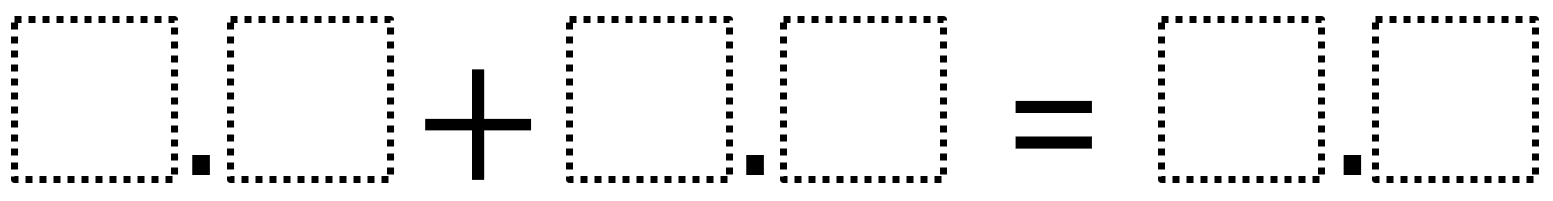Home > Grade 5 > Decimal Addition 2

# Decimal Addition 2

Directions: Use the digits 1 to 9, at most one time each, to make two true statements.### Hint

How do you place digits so the sum is less than ten?

### Answer

There are many answers including:
7.2 + 1.3 = 8.5
3.9 + 4.2 = 8.5
4.6 + 1.3 = 5.9

Source: Owen Kaplinsky

## Adding Three Fractions

Directions: Using each of the digits from 0-9 only once, fill in the boxes to …

### 2 comments

1.My fifth grade student was super proud she found 9 ways so far to complete this! She wanted me to post it here . Thanks!

2.Vedaant 4th Grade

I found out 20 ways to do this problem! Here are some ways:

4.8+3.1=7.9

3.2+4.7=7.9

7.1+2.3=9.4

1.5+2.4=3.9

8.3+1.4=9.7